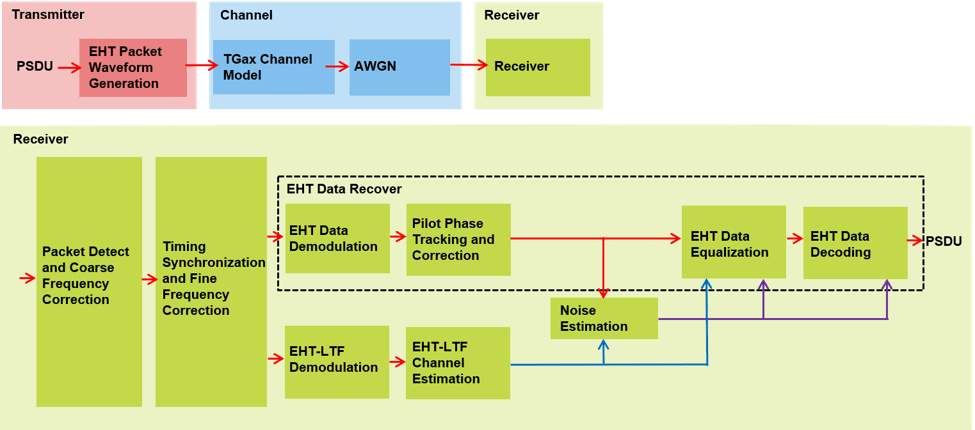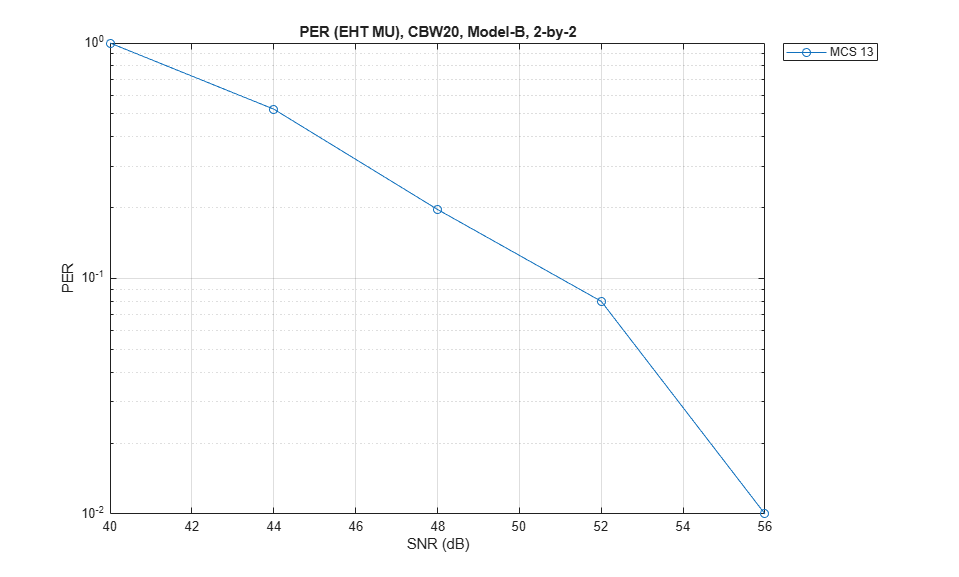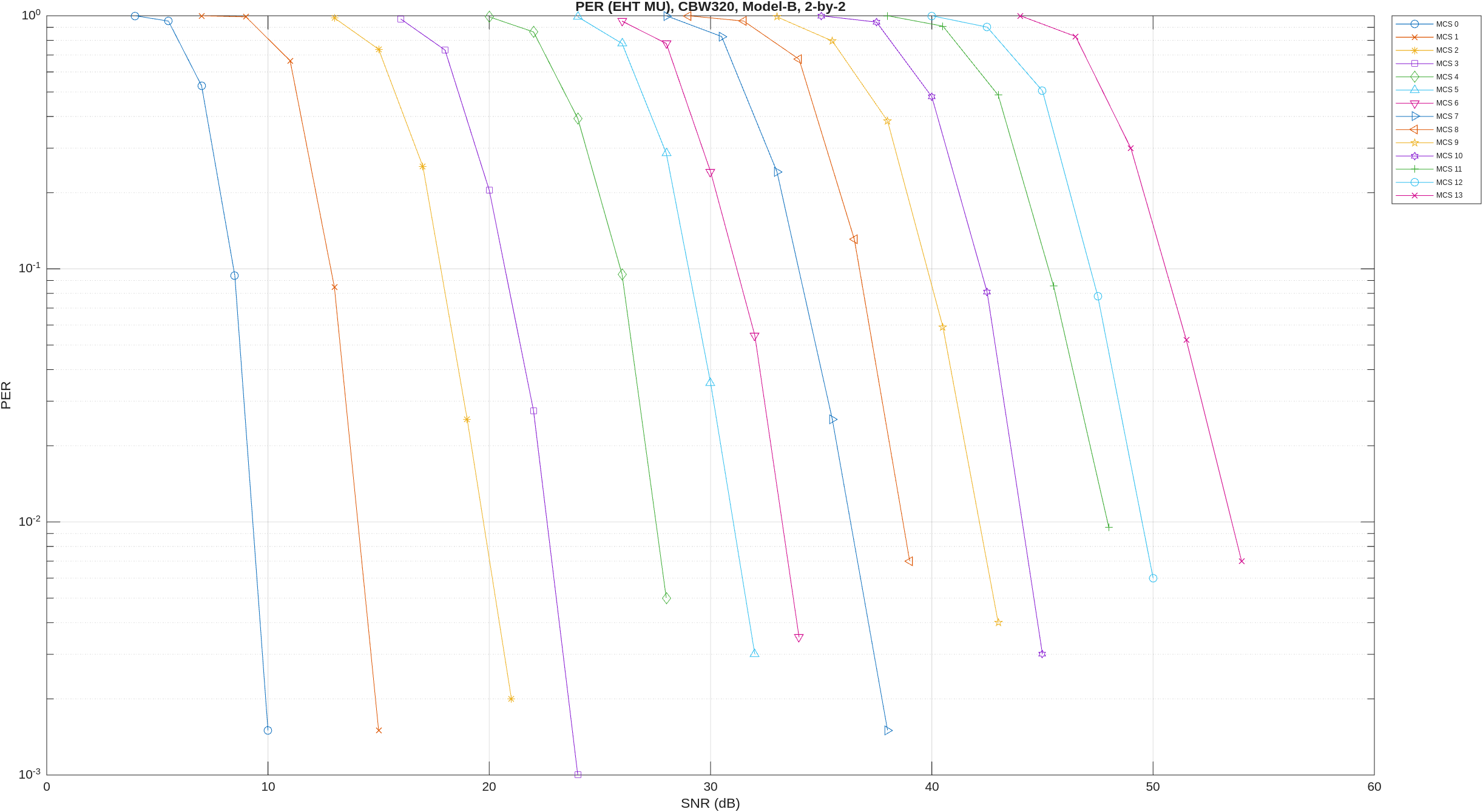# 802.11be Packet Error Rate Simulation for an EHT MU Single-User Packet Format

This example shows how to measure the packet error rate of an IEEE® 802.11be™ Extremely High Throughput multi-user (EHT MU) packet format link with a single user.

### Introduction

This example determines the packet error rate for an 802.11be [ 1 ] single-user (SU) link by using an end-to-end simulation for a selection of signal-to-noise ratio (SNR) points. At each SNR point, the example simulates the transmission of multiple packets through a noisy TGax indoor channel, then demodulates the received packets and recovers the PSDUs. The example then compares the transmitted and received packets to determine the packet error rate. This diagram shows the processing steps for each packet.### Waveform Configuration

An EHT MU SU packet is a full-band transmission to a single user. Configure the transmission parameters for an SU packet format by using the `ehtMUConfig` object. The properties of the object contain the physical layer (PHY) configuration.

Create a configuration object for an EHT MU transmission, setting a channel bandwidth of 20 MHz, an APEP length of 1000 bytes, two transmit antennas, two space-time streams, and a modulation and coding scheme (MCS) value of 13, which specifies 4096-point quadrature amplitude modulation (4096-QAM) and a coding rate of 5/6. If you specify `mcs` as a vector, the example performs the simulation for each MCS index value.

```chanBW = 'CBW20'; % Channel bandwidth cfgEHT = ehtMUConfig(chanBW); cfgEHT.User{1}.APEPLength = 1e3; % APEP length (bytes) numTx = 2; % Number of transmit antennas numRx = 2; % Number of receive antennas cfgEHT.NumTransmitAntennas = numTx; cfgEHT.User{1}.NumSpaceTimeStreams = numTx; % Number of space-time streams mcs = 13; % MCS index ```

### Channel Configuration

This example uses a TGax non-line-of-sight (NLOS) indoor channel model with delay profile Model-B. Model-B is considered NLOS when the distance between transmitter and receiver is greater than or equal to 5 meters. For more information about the TGax channel model, see `wlanTGaxChannel`.

```% Create and configure a 2x2 MIMO channel. tgaxChannel = wlanTGaxChannel; tgaxChannel.DelayProfile = 'Model-B'; tgaxChannel.NumTransmitAntennas = cfgEHT.NumTransmitAntennas; tgaxChannel.NumReceiveAntennas = numRx; tgaxChannel.TransmitReceiveDistance = 5; % Distance in meters for NLOS tgaxChannel.ChannelBandwidth = chanBW; tgaxChannel.LargeScaleFadingEffect = 'None'; fs = wlanSampleRate(chanBW); tgaxChannel.SampleRate = fs; ```

### Simulation Parameters

For each SNR point in `snrRange`, the example generates the specified number of packets, passes the packets through a channel, then demodulates the received signal to determine the packet error rate. Set the SNR values in the `snrRange` parameter to simulate the transition from all packets being decoded in error to all packets being decoded successfully as the SNR value increases for MCS 13. If you specify `snrRange` as a matrix, each row represents the SNR points for the corresponding MCS index, defined in `mcs`.

```snrRange = 37:5:57; % Set the range of SNR values ```

These parameters control the number of packets tested for each SNR point.

1. `maxNumErrors`: the maximum number of packet errors simulated for each SNR point. When the number of packet errors reaches this limit, the simulation at this SNR point is complete.

2. `maxNumPackets`: the maximum number of packets simulated for each SNR point, which limits the length of the simulation if the simulation does not reach the packet error limit.

The default parameter values lead to a very short simulation. For meaningful results, increase these values.

```maxNumErrors = 10; maxNumPackets = 100; ```

### Processing SNR Points

This section measures the packet error rate for each SNR point by performing these processing steps for the specified number of packets.

1. Create a PSDU and encode to generate a single-packet waveform.

2. Pass the waveform through an indoor TGax channel model, using different channel realizations for each packet.

3. Add AWGN to the received waveform to create the desired average SNR per subcarrier after OFDM demodulation. The configuration accounts for the normalization within the channel by the number of receive antennas and the noise energy in unused subcarriers. The example removes the unused subcarriers during OFDM demodulation.

4. Detect the packet

5. Estimate and correct coarse carrier frequency offset (CFO)

6. Perform fine timing synchronization by using L-STF, L-LTF, and L-SIG samples. This synchronization enables packet detection at the start or end of the L-STF.

7. Estimate and correct fine CFO

8. Extract the EHT-LTF from the synchronized received waveform

9. OFDM demodulate the EHT-LTF and perform channel estimation

10. Extract the data field from the synchronized received waveform and perform OFDM demodulation

11. Track any residual CFO by performing common phase error pilot tracking

12. Perform noise estimation by using the demodulated data field pilots and single-stream channel estimation at pilot subcarriers

13. Equalize the phase corrected OFDM symbols by using channel estimation

14. Recover the PSDU by demodulating and decoding the equalized symbols

This example also demonstrates how to speed up simulations by using a `parfor` loop instead of a `for` loop when simulating each SNR point. The `parfor` function executes processing for each SNR in parallel to reduce the total simulation time. Use a `parfor` loop to parallelize processing of the SNR points. To use parallel computing for increased speed, comment out the `for` statement and uncomment the `parfor` statement in this code.

```numSNR = size(snrRange,2); % Number of SNR points numMCS = numel(mcs); % Number of MCS packetErrorRate = zeros(numMCS,numSNR); for imcs = 1:numel(mcs) cfgEHT.User{1}.MCS = mcs(imcs); ofdmInfo = ehtOFDMInfo('EHT-Data',cfgEHT); % SNR points to simulate from MCS snr = snrRange(imcs,:); ind = ehtFieldIndices(cfgEHT); %parfor isnr = 1:numSNR % Use parfor to speed up the simulation for isnr = 1:numSNR % Use for to debug the simulation % Set random substream index per iteration to ensure that each % iteration uses a repeatable set of random numbers stream = RandStream('combRecursive','Seed',99); stream.Substream = isnr; RandStream.setGlobalStream(stream); % Define the SNR per active subcarrier to account for noise energy % in nulls snrValue = snr(isnr)-10*log10(ofdmInfo.FFTLength/ofdmInfo.NumTones); % Loop to simulate multiple packets numPacketErrors = 0; numPkt = 1; % Index of packet transmitted while numPacketErrors<=maxNumErrors && numPkt<=maxNumPackets % Generate waveform txPSDU = randi([0 1],getPSDULength(cfgEHT)*8,1); % PSDULength (bytes) tx = ehtWaveformGenerator(txPSDU,cfgEHT); % Add trailing zeros to allow for channel delay txPad = [tx; zeros(50,cfgEHT.NumTransmitAntennas)]; % Pass through fading indoor TGax channel reset(tgaxChannel); % Reset channel for different realization rx = tgaxChannel(txPad); % Pass waveform through an AWGN channel rx = awgn(rx,snrValue); % Detect packet and determine coarse packet offset coarsePktOffset = wlanPacketDetect(rx,chanBW); if isempty(coarsePktOffset) % If empty no L-STF detected, packet error numPacketErrors = numPacketErrors+1; numPkt = numPkt+1; continue; % Go to next loop iteration end % Extract L-STF and perform coarse frequency offset correction lstf = rx(coarsePktOffset+(ind.LSTF(1):ind.LSTF(2)),:); coarseFreqOff = wlanCoarseCFOEstimate(lstf,chanBW); rx = helperFrequencyOffset(rx,fs,-coarseFreqOff); % Extract the non-HT fields and determine fine packet offset nonhtfields = rx(coarsePktOffset+(ind.LSTF(1):ind.LSIG(2)),:); finePktOffset = wlanSymbolTimingEstimate(nonhtfields,chanBW); % Determine final packet offset pktOffset = coarsePktOffset+finePktOffset; % If packet detected outwith range of expected delays from % the channel modeling, packet error if pktOffset>50 numPacketErrors = numPacketErrors+1; numPkt = numPkt+1; continue; % Go to next loop iteration end % Extract L-LTF and perform fine frequency offset correction rxLLTF = rx(pktOffset+(ind.LLTF(1):ind.LLTF(2)),:); fineFreqOff = wlanFineCFOEstimate(rxLLTF,chanBW); rx = helperFrequencyOffset(rx,fs,-fineFreqOff); % EHT-LTF demodulation and channel estimation rxHELTF = rx(pktOffset+(ind.EHTLTF(1):ind.EHTLTF(2)),:); heltfDemod = ehtDemodulate(rxHELTF,'EHT-LTF',cfgEHT); [chanEst,pilotEst] = ehtLTFChannelEstimate(heltfDemod,cfgEHT); % Demodulate the Data field rxData = rx(pktOffset+(ind.EHTData(1):ind.EHTData(2)),:); demodSym = ehtDemodulate(rxData,'EHT-Data',cfgEHT); % Perform pilot phase tracking demodSym = ehtCommonPhaseErrorTracking(demodSym,chanEst,cfgEHT); % Estimate noise power in EHT fields nVarEst = ehtNoiseEstimate(demodSym(ofdmInfo.PilotIndices,:,:),pilotEst,cfgEHT); % Extract data subcarriers from demodulated symbols and channel % estimate demodDataSym = demodSym(ofdmInfo.DataIndices,:,:); chanEstData = chanEst(ofdmInfo.DataIndices,:,:); % Equalization [eqSym,csi] = ehtEqualizeCombine(demodDataSym,chanEstData,nVarEst,cfgEHT); % Recover data field bits rxPSDU = ehtDataBitRecover(eqSym,nVarEst,csi,cfgEHT,1,'LDPCDecodingMethod','norm-min-sum'); % Determine if any bits are in error packetError = any(biterr(txPSDU,rxPSDU)); numPacketErrors = numPacketErrors+packetError; numPkt = numPkt+1; end % Calculate PER at SNR point packetErrorRate(imcs,isnr) = numPacketErrors/(numPkt-1); disp(['MCS ' num2str(mcs(imcs)) ','... ' SNR ' num2str(snr(isnr)) ... ' completed after ' num2str(numPkt-1) ' packets,'... ' PER:' num2str(packetErrorRate(imcs,isnr))]); end end ```
```MCS 13, SNR 37 completed after 11 packets, PER:1 MCS 13, SNR 42 completed after 11 packets, PER:1 MCS 13, SNR 47 completed after 20 packets, PER:0.55 MCS 13, SNR 52 completed after 63 packets, PER:0.1746 MCS 13, SNR 57 completed after 100 packets, PER:0.01 ```

### Plot Packet Error Rate vs SNR

```markers = 'ox*sd^v><ph+ox*sd^v><ph+'; color = 'bmcrgbrkymcrbmcrgbrkymcr'; figure; for imcs = 1:numMCS semilogy(snrRange(imcs,:),packetErrorRate(imcs,:).',['-' markers(imcs) color(imcs)]); hold on; end grid on; xlabel('SNR (dB)'); ylabel('PER'); dataStr = arrayfun(@(x)sprintf('MCS %d',x),mcs,'UniformOutput',false); legend(dataStr,'Location','NorthEastOutside'); title(['PER (EHT MU), ' num2str(cfgEHT.ChannelBandwidth) ', Model-B, ' num2str(numTx) '-by-' num2str(numRx)]); ```### Further Exploration

The `maxNumErrors` and `maxNumPackets` parameters control the number of packets tested for each SNR point. For meaningful results, increase these values. For example, this figure shows results for a channel bandwidth of 320 MHz, an APEP length of 16000 bytes, MCS values of 0-13, a `maxNumErrors` value of 100, and a `maxNumPackets` value of 1000. The corresponding SNR values for MCS between 0 and 13 are:

```snrRange = [... 8:1:13; ... % MCS 0 8:2:18; ... % MCS 1 16:2:26; ... % MCS 2 18:2:28; ... % MCS 3 24:2:34; ... % MCS 4 26:2:36; ... % MCS 5 28:2:38; ... % MCS 6 32:2:42; ... % MCS 7 34:2:44; ... % MCS 8 36:2:46; ... % MCS 9 38:2:48; ... % MCS 10 42:2:52; ... % MCS 11 44:2:54; ... % MCS 12 45:3:60]; ...% MCS 13 ```### Selected Bibliography

1. IEEE Std 802.11be™/D1.0 Draft Standard for Information technology - Telecommunications and information exchange between systems Local and metropolitan area networks - Specific requirements - Part 11: Wireless LAN Medium Access Control (MAC) and Physical Layer (PHY) Specifications. Amendment 8: Enhancements for Extremely High Throughput (EHT).

## SupportGet trial now